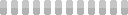physics > Questions and Answers > BMEN3350 EXAM 1 Solution

# BMEN3350 EXAM 1 Solution

Problem 1 (20 points): #Q1. (3 points) A 14-bit analog-to-digital converter (ADC) has an input range of 1V to 6 V. If the output of the ADC is 74, what is the voltage at its input? Ignore quantization error. 6−1 74× 14 +1=1.02258 V 2 −1#Q1. (3 points) A 12-bit analog-to-digital converter ( ... ADC) has an input range of 2V to 5 V. If the output of the ADC is 47, what is the voltage at its input? Ignore quantization error. 6−15−2 47× +2= 2.0344 V 212 −1 #Q2. (3 points) Briefly state the purpose of using voltage followers. Used as a buffer between two stages since it has infinite input impedance and zero output impedance. #Q3. (3 points) An analog signal contains three sinusoidal components with frequencies 5, 7 and 12 Hz. What is the minimum sampling rate required in order to avoid aliasing? 24 sps #Q3. (3 points) An analog signal contains three sinusoidal components with frequencies 5, 7 and 24 Hz. What is the minimum sampling rate required in order to avoid aliasing? 48 SPS 1 [Show More]

##### Preview: 4 out of 16 pagesGenerating document previews ...

#### Purchase the document to get the full access instantly

Trusted by 100,000+ Students
100% Money Back Guarantee
Immediately available after payment

Price:

#### \$8.50

Pages:

16 pages

Rating:

88
0master papers
Joined: 2 years ago
Papers sold: 244

##### Written for

Category:

Course:

physics

Last updated at :

2 months ago

Language
English